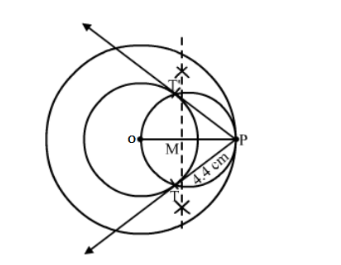# Draw two concentric circles of radii 4 cm and 6 cm.`
Question:

Draw two concentric circles of radii 4 cm and 6 cm. Construct a tangent to the smaller circle from a point on the larger circle. Measure the length of this tangent.

Solution:

Steps of Construction :

Step 1. Draw a circle with O as centre and radius 6 cm.
Step 2. Draw another circle with O as centre and radius 4 cm.
Step 2 . Mark a point P on the circle with radius 6 cm.
Step 3. Join OP and bisect it at M.
Step 4. Draw a circle with M as centre and radius equal to MP to intersect the given circle with radius 4 cm at points T and T'.
Step 5. Join PT and P T'.Thus, PT or P T'are the required tangents and measure 4.4 cm each.